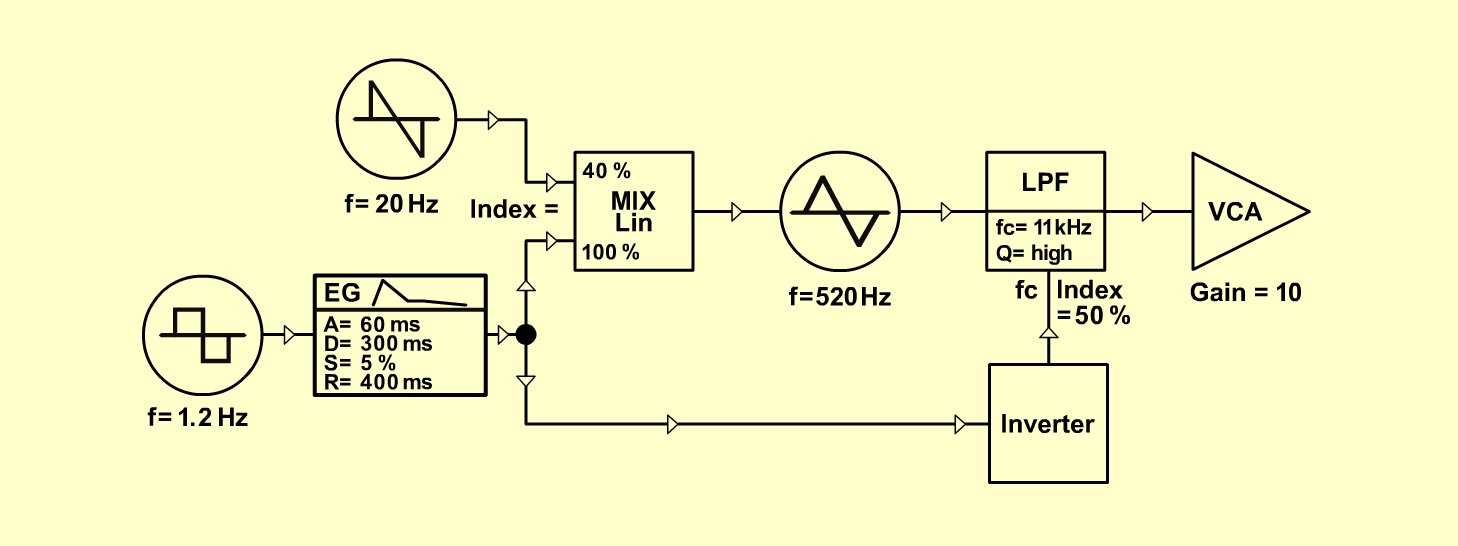EMS 01FXD o e p f e r A - 100 Connections: Settings: A-145/1 (Pulse)  <=>  A-140 (Gate) A-140 (Output)  <=>  A-111 (CV1) A-145/2 (Saw Down)  <=>  A-111 (CV2) A-111 (Triangle)  <=>  A-120 (Audio In) A-140 (Inverse Out)  <=>  A-120 (CV3) A-120 (Audio Out)  <=>  A-131 (Audio In) A-131 (Audio Out)  <=> Amplifier A-111 (Octave = +3, Tune = 5, Fine = 5, CV 2 = 4) A-120 (Freq = 8.5, CV3 = 5, Lev = 8, Res = 8) A-140 (A = 2, D = 5, S = 2, R = 7.5, Range = M) A-145/1 (Frq = 2.5, Range = M) A-145/2 (Frq = 7, Range = M) A-131 (Gain = 10, Audio In 1 = 10, Audio Out = 10) Notes: Pitch A-111: 520 Hz. A-111: The VCO A-110 is also possible. Axel Jungkunst, April 2001Sound sample Ems 01